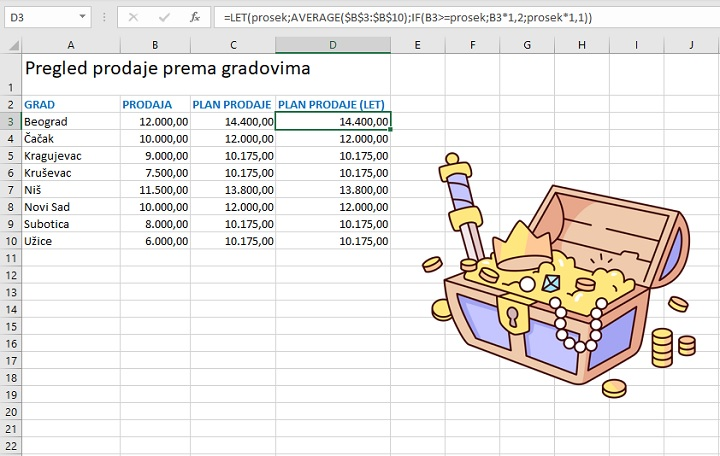# LET function

The LET command exists in several programming languages ​​and is used to assign values ​​to variables. In a similar way in Excel, you can declare one or more variables, assign values ​​to them, and define the expression in which they are used using the LET function. This is especially beneficial when there are parts within the formula that are repeated several times. The LET function, in addition to shortening the formula, also significantly affects the performance …

The syntax of this function is:

LET(<variable 1>;<value 1>…<variable n>;<value n>;<expresion>)

The simplest example is when we have one variable:

=LET(x,2,x^2)

In this formula, we declare the variable x and then calculate its square. The formula returns the number 4.

What would a formula with two variables look like?

=LET(x,1,y,2,x+y)

Here we declared x, gave it the value 1, and then y which got the value 2. Finally, we wrote an expression that represents the sum of x and y. The formula returns number 3.

This was a bit of a theory, let’s see how we can use the LET function in practice. Imagine the following scenario: we are looking at a sales report by cities and we want to make a sales plan for next month. Criterion: if sales were greater than or equal to average sales, the plan should be the value of sales increased by 20%, and if it was lower it should be the average value of sales increased by 10%. We perform the calculation using the formula:

=IF(B3>=AVERAGE(\$B\$3:\$B\$10),B3*1.2,AVERAGE(\$B\$3:\$B\$10)*1.1)

The formula is a little clumsy, isn’t it? To simplify and speed up the calculation, we will use the LET function:

=LET(prosek,AVERAGE(\$B\$3:\$B\$10),IF(B3>=prosek,B3*1.2,prosek*1.1))

As you can see, the formula is much more elegant, and the average is calculated only once, which makes it twice as fast.